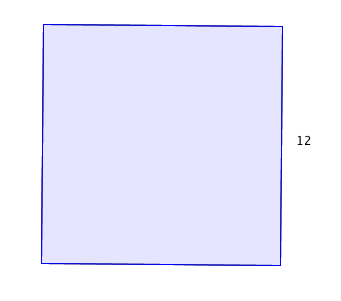Everyday Math

# Area - Rectangles

What is the area (in m$^2$) of a rectangle with side length 5 m and width 15 m?

Benny drew a square with side length 12. What is the area of the square?Far Eastern school has a rectangular field of area 3500 m$^2$. If the width of the field is $50$ m, what is the length (in meters) of the field?

A rectangle has length 17 meters and area 136 meters$^2$. What is the perimeter (in meters) of the rectangle?

Calvin's monitor screen measures 8 inches by 13 inches. What is the area of his screen (in inches$^2$)?

×